## RD Sharma Class 9 Solutions Chapter 6 Factorisation of Polynomials Ex 6.3

These Solutions are part of RD Sharma Class 9 Solutions. Here we have given RD Sharma Class 9 Solutions Chapter 6 Factorisation of Polynomials Ex 6.3

Other Exercises

In each of the following, using the remainder Theorem, find the remainder when f(x) is divided by g(x) and verify the result by actual division (1 – 8) :

Question 1.
f(x) = x3 + 4x2 – 3x + 10, g(x) = x + 4
Solution: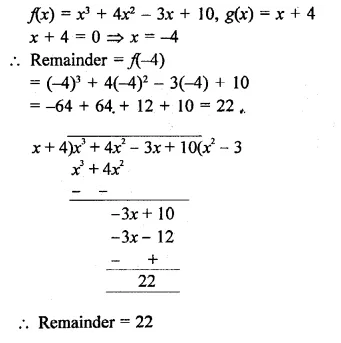Question 2.
f(x) – 4x4 – 3x3 – 2x2 + x – 7, g(x) = x1
Solution: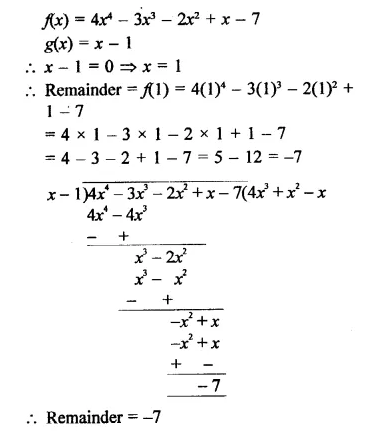Question 3.
f(x) = 2x4 – 6X3 + 2x2 – x + 2, ,g(x) = x + 2
Solution:Question 4.
f(x) = 4x3 – 12x2 + 14x – 3, g(x) = 2x – 1
Solution: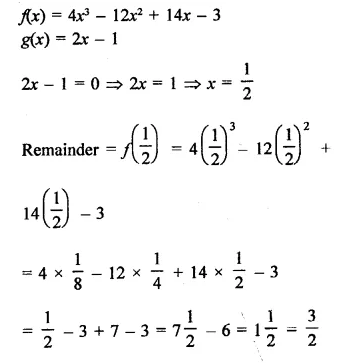Question 5.
f(x) = x3 – 6x2 + 2x – 4, g(x) = 1 – 2x
Solution: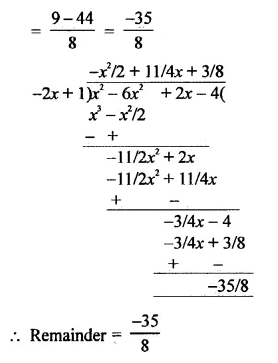Question 6.
f(x) = x4 – 3x2 + 4, g(x) = x – 2
Solution: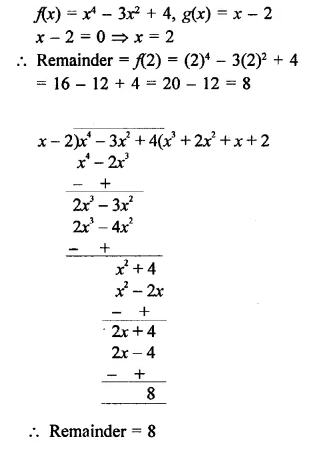Question 7.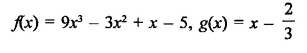Solution: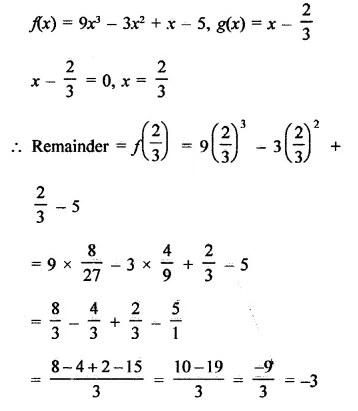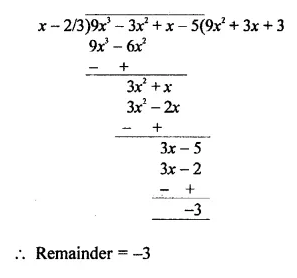Question 8.Solution: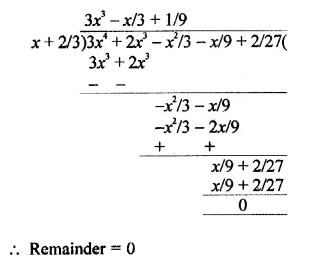Question 9.
If the polynomials 2x3 + ax2 + 3x – 5 and x3 + x2 – 4x + a leave the same remainder when divided by x – 2, find the value of a.
Solution:
Let f(x) = 2x3 + ax2 + 3x – 5
g(x) = x3+x2-4x + a
q(x) = x –  2 ⇒ x-2 = 0  ⇒x = 2
∴ Remainder =f(2) = 2(2)3 + a(2)2 + 3 x 2-5
= 2 x 8 4-a x 4 + 3 x 2-5
= 16 + 4a + 6 – 5
= 4a +17
and g(2) = (2)3 + (2)2 -4×2 + a
= 8 + 4 – 8 + a = a + 4
∵  In both cases, remainder are same
∴  4a + 17 = a + 4
⇒  4a – a = 4 – 17 ⇒  3a = -13
⇒ a = $$\frac { -13 }{ 3 }$$
Hence a = $$\frac { -13 }{ 3 }$$

Question 10.
If the polynomials ax3 + 3x2 – 13 and 2x3 – 5x + a, when divided by (x – 2), leave the same remainders, find the value of a.
Solution:
Let p(x) = ax3 + 3x2 – 13
q(x) = 2x5x + a
and divisor g(x) = x – 2
x-2 = 0
⇒ x = 2
∴ Remainder = p(2) = a(2)3 + 3(2)2 – 13
= 8a + 12 – 13 = 8a – 1
and q( 2) = 2(2)3 – 5×2 + a=16-10 + a
= 6 + a
∵  In each case remainder is same
∴ 8a – 1 = 6 + a
8a – a = 6 + 1
⇒  7a = 7
⇒ a = $$\frac { 7 }{ 7 }$$= 1
∴ a = 1

Question 11.
Find the remainder when x3 + 3x2 + 3x + 1 is divided by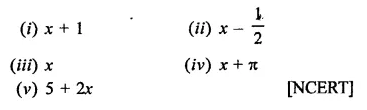Solution: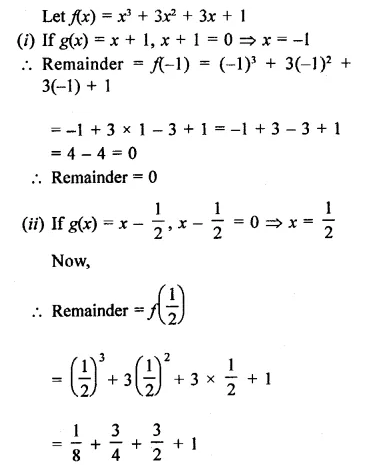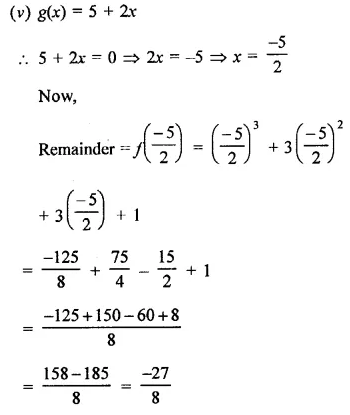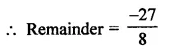Question 12.
The polynomials ax3 + 3a-2 – 3 and 2x3 – 5x + a when divided by (x – 4) leave the remainders R1 and R2, respectively. Find the values of a in each case of the following cases, if
(i) R1 = R2
(ii) R1 + R2 = 0
(iii) 2R1 – R2 = 0.
Solution: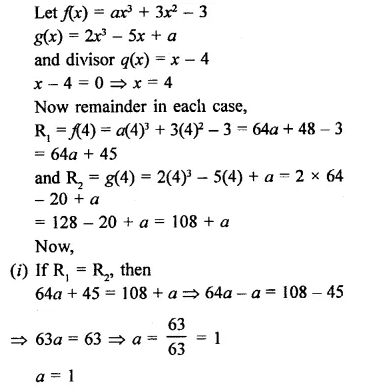Hope given RD Sharma Class 9 Solutions Chapter 6 Factorisation of Polynomials Ex 6.3 are helpful to complete your math homework.

If you have any doubts, please comment below. Learn Insta try to provide online math tutoring for you.